# Multi-Layer Co-Occurrence Matrices for Person Identification from ECG Signals

Multi-Layer Co-Occurrence Matrices for Person Identification from ECG Signals
Electrical and Electronics Engineering, Siirt University, Siirt 56100, Turkey

Computer Engineering, Siirt University, Siirt 56100, Turkey

Corresponding Author Email:
melihkuncan@siirt.edu.tr
Page:
431-440
|
DOI:
https://doi.org/10.18280/ts.390204
22 February 2022
|
Accepted:
3 April 2022
|
Published:
30 April 2022
| Citation

OPEN ACCESS

Abstract:

Recently, numerous researches have been executed to create reliable systems to recognize persons based on their biometric information. As a result, person identification (PI) systems have become popular among researchers using different methods. In recent years, it is seen that Electrocardiogram (ECG) signals have started to be used for biometric systems as well as health-related studies. Because ECG data is unique for each person cannot be imitated or copied for biometric studies, it is advantageous for PI problems compared to other biometric data. In this study, we have conducted a method that uses One Dimensional Multi-Layer Co-Occurrence Matrices (1D-MLGLCM) to recognize individuals based on their ECG signals. The dataset used in the experiments contains ECG data of 90 subjects whose ages ranged from 13 to 75 years. First of all, ECG signals are normalized at 32 different intervals for the PI system. Then, Dimensional Co-Occurrence Matrices (1D-GLCM) are applied to each signal to construct co-occurrence matrices. These matrices are used to extract Heralick features to feed classification algorithms such as Random Forest (RF), Support Vector Machine (SVM), Naive Bayes (NB), Bayes Net (BN), and K-Nearest Neighborhood (KNN). Our proposed method achieved a 93.414% success rate by using SVM. As a result, the study proves that the suggested method has achieved very effective outcomes by using ECG signals for person identification problems.

Keywords:

GLCM, 1D-GLCM, 1D-MLGLCM, feature extraction, ECG, person identification

1. Introduction

In recent years, it has been observed that computer-based signal processing studies have been successfully applied in many fields. The primary purpose of signal processing is to obtain information or estimation from signals using machine learning techniques. For this reason, researchers have a particular interest in this research area to extract meaningful information from medical signals. Today, it has been observed that medical signals are widely used in many different disciplines (medicine, sports, security, etc.). Technical developments, especially in electronics and computer science, offer opportunities for interdisciplinary work in all fields of science and medical science. Bio-Electrical signals provide us with crucial information about medical science. The measurement phase of the Bio-Electrical signals formed in the human body can be easily transferred through the skin with electrodes consisting of the following parts: measuring object (human), electrodes, amplifier, filter, and display device, respectively . There are many types of Bio-Electrical Signals in medical science. Researchers commonly use Electroencephalography (EEG), Electromyography (EMG), and Electrocardiogram (ECG) signals in medical analysis.

Voltage changes can be measured to track heart movements in a human. The signal that occurs when the heart is beating is the most significant amplitude signal transmitted through the skin. In medical diagnosis, it is impossible to reach the "current sources" in the body directly, so action potentials that can be transferred through the skin have to be contented with. The electrical activity signals of the heart are called electrocardiograms (ECG). Researchers are widely conducting medical studies using ECG signals. The primary purpose of these studies is to obtain helpful information about the diagnosis of a disease or the health status of any person with different machine learning methods using ECG signals. Because it is known that medical signals provide remarkable features for biometric studies, machine learning methods have been successfully implemented for person identification, age detection, and gender recognition problems [2-4]. Many biometric methods have been successfully used to authenticate individuals in a multitude of scenarios reliably. However, very high dimensional data are needed to achieve successful results in data fields such as iris, fingerprint, and walking process. One of the challenges in this field, especially in video-based biometrics, is achieving high accuracies. Recently, some studies have reported that the bioelectrical signals of the brain (EEG), muscles (EMG), and heart (ECG) are unique for each person (uniqueness discrimination). Amongst all of these unseen human actions, ECG-based recognition has received much attention lately. Given that this interest is relatively tricky, its constant accessibility has led to the argument of privacy concerns for using it as an alternative biometric data for personal identification. At the same time, ECG is concluded to be a decisive practice compared to the current methods used for PI systems [5-7].

The PI problem has become one of the famous research areas in computer science that researchers have used machine learning methods in recent years. Researchers have generally worked in this area using the person's characteristics such as retina, fingerprint, and voice. Although face, fingerprint, palm print, and voice recognition techniques have been used successfully in PI, a single biometric feature may not be sufficient to authenticate a person. PI systems that rely on information from a single sensor have weaknesses. These systems increase the rate of encountering uncertainty while the number of people increases. Multi-mode modules may perform better than single-mode systems because they can provide complementary information . For example, among a large population, two or more people may have similar faces, especially under changes in appearance due to changes in expression, lighting condition, and exposure to the subject. However, it can be determined that they do not exhibit similar behaviors when walking, movement, and mimics are involved. However, studies in the field of ECG-based biometrics have shown that ECG-based PI studies are not very common at this time [9, 10].

In this study, PI was performed by the 1D-MLGLCM method using ECG signals. The open-source dataset provided by Physionet was used in the study. The dataset includes ECG data of 90 volunteers (46 female and 44 male) whose ages ranged from 13 to 75. ECG signal from each individual has been normalized and spliced into 32 different smaller parts. Those small parts are processed by the 1D-GLCM method to create co-occurrence matrices. These matrices are necessary to extract Heralick features. Once the features are extracted, they can be fed into RF, SVM, NB, BN, and SVM classification algorithms. The proposed technique achieves a 93.414% accuracy rate with the SVM classification method. This study proves that using ECG data to recognize individuals is a highly efficient technique. Thanks to our study, it has been analyzed that the novel method suggested by using ECG signals has achieved high success in-person identification. Also, the method, as foreseen, can be applied in different intelligent systems. Moreover, it can be used safely in various problems.

The remainder of this study is as follows. In the next section, literature on PI from ECG signals will be given. In the 3rd section, the data set is explained in detail. In Section 4, the proposed feature extraction method, 1D-MLGLCM, and classification algorithms are explained. The results obtained in the 5th section are given in detail. The results are discussed in the last section.

2. The Related Studies

Studies using medical signals have been a vital research scope in recent years. Sensor-based medical signals are obtained using different devices attached to the human body. These sensors aim to capture the status of the human and the environment by continuously monitoring very physiological signals that project the status of a person’s activities. Particularly in the last few years, it has been monitored that computer-based diagnosis systems have vitally developed using medical devices and mobile devices in medical signal applications.

Electroencephalogram (EEG), electromyography (EMG), and electrocardiogram (ECG) data came to the fore as the types of signals obtained in medical-based studies and studied extensively. Machine learning methods provide us to solve person identification, age detection, and gender recognition problems using medical signals. Because it is known that medical signals have a distinguishing aspect for biometric studies. Many biometric methods have been successfully used to authenticate individuals in a multitude of scenarios reliably. In the last ten years, it has been seen that some researchers have been using the ECG signal to identify people (personal identification).

Chan et al.  presented a new biometric evaluation study based on electrocardiogram (ECG) waveforms. ECG data were collected from 50 people during three data recording sessions on different days using a simple user interface. Using the thumbs and index fingers of the subjects, the data was obtained by holding the two electrodes on the pads of their thumbs. The data obtained in the first session were used to create a registered database, and the data obtained from the remaining two sessions were used as test scenarios. The classification was carried out using three different features. First, new distance measurement methods were chosen based on percent residual difference, correlation coefficient, and wavelet transform. The authors stated that they achieved a classification accuracy of 89% with the measure of wavelet distance.

Pathoumvanh et al.  stated in their study that electrocardiogram (ECG) signals could be used as a biometric system. In their study, the authors received a single leaded average ECG signal from ten people firstly. Later, each acquired pulsed ECG data is segmented and analyzed in the Continuous Wavelet Transform (CWT) domain. The total energy of the wavelet coefficients was calculated for each P, QRS, and T segment. Then Fisher Linear Discriminant Analysis (FLDA) was applied. The authors stated in their experimental results that they achieved a classification accuracy of 97% in a standard ECG condition (without change in heart rate).

Waili et al.  used an electrocardiogram (ECG) to obtain individuals' identity, mood, and behavior. In an ECG signal, the region defined as the QRS complex was primarily used to classify individuals. In their experiments, the authors measured the processing time to study the variety of feature points and the speed of processing feature points for identification. The authors presented a new method for using the 3-point QRS complex, which can provide the best accuracy and time performance in classification. Although the accuracy results of the proposed method are not very good, it gives faster results. They stated that the proposed method can be used for future applications in IoT.

Bassiouni et al.  presented a machine learning technique for person identification using electrocardiograms (ECG). The proposed technique consists of four processes. These processes are expressed as data collection, preprocessing, feature extraction, and classification. In the first step, the dataset was collected from the MIT-BIH Arrhythmia database running on 30 subjects using lead II (MLII) obtained by placing the electrodes on the chest. They stated that the second step is concerned with reducing noise on the ECG by eliminating baseline drift, power line interference, and high-frequency noise. Finally, the feature extraction process is applied using a non-reference approach based on autocorrelation and discrete cosine transform (AC / DCT). As a result of the study, the authors mentioned that they achieved a classification precision of 97%.

Matveev et al.  investigated the potential of a series of ECG morphological features for person verification/identification. ECG measurements were performed on 145 pairs of ECG records taken from healthy subjects over a five-year period. The authors stated that the results obtained in their study showed that, in general, the morphological features measured from the electrocardiogram can be applied to identify subjects at different examination periods.

Sulam et al.  proposed a generic classification and identification framework based on a multi-level learning algorithm. The suggested approach was tested on a synthetic sample before being applied to the person identification problem using ECG recordings. The authors noted that their approach achieved a recognition accuracy of 97.25%, the highest accuracy for the database they were testing (on a database of 90 subjects).

Brás et al.  provided an innovative and robust solution for biometric and emotion identification using an electrocardiogram (ECG). They stated that the ECG represents the electrical signal coming from the contraction of the heart muscles and indirectly represents the blood flow in the heart, carrying information that allows biometric identification. They stated that depending on their relationship with the nervous system, it also changed as a function of their emotional state. The authors have stated the proposed method in three steps. They are defined as; (1) Converting the true-valued ECG recording into a symbolic time series using a quantization process; (2) conditional compression of the symbolic representation of the ECG using symbolic ECG records stored in the database as a reference; (3) Determination of ECG recording class using a 1-NN (nearest neighbor) classifier. When the results were examined, the authors stated that they achieved an accuracy of over 98% in biometric identification and success of over 90% in emotion recognition.

Truong et al.  stated in their study that single-mode identification systems are prone to errors in the collection of sensor data, and as a result, they are more prone to misidentify people. For example, they have stated that relying only on an RGB face camera data can cause problems in poorly lit environments or if subjects are not facing the camera. They stated that other identification methods such as electrocardiograms (ECG) have problems with electrode connections that are unsuitable for the skin. The authors stated that errors in identification could be minimized by combining information gathered from both of these models. The authors proposed a methodology for combining face and ECG data identification results using Part A of the BioVid Heat Pain Database, which contains synchronized RGB-video and ECG data on 87 subjects. They stated that when 10-fold cross-validation was used, facial recognition was 98.8% accurate, while ECG identification was 96.1% correct. The authors stated that they increased the identification accuracy to 99.8% by using a fusion approach. They noted that the proposed method allows distinct face and ECG models with non-overlapping modalities to improve identification accuracy considerably.

Su et al.  stated in their study that biometric human identification means the automatic recognition of individuals according to their biological or behavioral characteristics. They stated that finger vein recognition and electrocardiogram (ECG) recognition studies had attracted significant attention for more than a decade. These two features are seen as promising biometric features due to their unique advantages. However, these two independent single-mode biometrics, which use a single biometric feature for personal recognition, have often stated that they cannot fulfill the criteria of real-world applications. Therefore, the authors tried to integrate the finger vein with ECG signals for personal identification using various fusion strategies. They proposed a new multimodal biometric method based on Discriminant correlation analysis (DCA) to fuse finger vein and ECG. They conducted extensive experiments on the combined bimodal dataset named VeinECG from the FVPolyU finger vein dataset and the ECG-ID Dataset. The author's experimental results indicated that the proposed multi-mode system is significantly superior to two separate single-mode systems in terms of recognition accuracy and security.

Benouis et al.  proposed a developed version of the 1-dimensional local binary pattern model to derive the most suitable features for ECG-based human recognition. In general, they noted that by its nature, ECG signal characteristics present some significant problems, mostly related to sensitivity to sounds, behavioral and emotional disorders, and other factors of variability. To overcome this critical problem, they used the One Dimensional Local Difference Model (1D-LDP) operator to extract distinctive statistical features from the ECG using the difference between consecutive neighboring samples to capture both micro and macro pattern information in a heartbeat. The authors stated that they performed the classification models with K-nearest neighbors (KNN), linear support vector machine (SVM), and neural network. The authors stated that they achieved 93.33% success as a result of the analysis studies. In addition, the author(s) mentioned that the results obtained outperformed the 1D-LDP operator than the 1D-LBP variants available in the MIT-BIH Normal Sinus Rhythm and ECG-ID database .

3. Data Set

## 1.png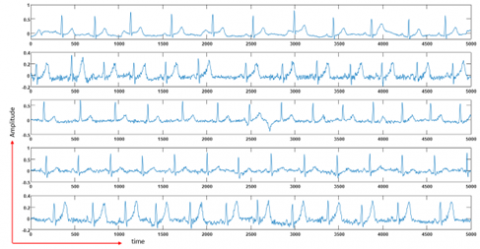Figure 1. ECG signal samples from different people

A detailed study of the ECG dataset was performed by Morone et al. . Lugovaya  created the dataset to use for her graduate research and granted open access to other researchers. In the dataset, there are a total of 310 records taken from 90 different people. Signals are 20 seconds long. These received signals were digitized with 10mV nominal amplitude, 12-bit resolution, and 500Hz frequency. Volunteers, whose ECG signals were measured, consisted of 44 males and 46 females aged between 13 and 75 years. At least two and at most 20 records were taken for each person. Both the raw and the noise-free filtered versions of the recorded signals are available at physionet.org. Sample signal parts belonging to random subjects from the dataset are given in Figure 1.

4. Method

4.1 Gray level co-occurrence matrix

The Gray Level Co-Occurrence Matrix (GLCM) method has been proposed by Heralik and Shanmugam  for classifying different textures in image processing. The GLCM is a pixel-based image processing technique. This method is a practice that uses the relationship between pixels to obtain a feature from a gray-level image. Forming of the GLCM matrix; the distance between pixels (D), the angle of the pixels (0º, 45º, 90º, and 135º = θ), and the number of grayscale levels to which the transformation will be performed (maximum 256) are based on the parameters. In this GLCM technique, primarily, the image is rescaled according to the determined number of grayscale levels. The number of neighboring pixels at the specified angle and distance in the rescaled image with the specified gray tone is assigned to the created GLCM matrix. The GLCM technique is used differently for feature extraction from one-dimensional (1D) signals in that work. Firstly, the ECG signals have been converted into values between 0-255. Then, the conversion process is carried out with the following equation.

$\operatorname{New} X_{i}=\operatorname{round}\left(\left[\frac{X_{i}-\operatorname{Min}(X)}{\operatorname{Max}(X)-\operatorname{Min}(X)}\right] x 255\right)$      (1)

The Co-Occurrence Matrix is calculated from the newly formed signals. In the new proposed approach, there is distance information rather than angle information. The application technique of the proposed method is explained as follows. Suppose Figure 2(A) is a signal showing four different pattern values between 0 and 3. The co-occurrence matrix for a sample signal is calculated as in Figure 2(B). # (i, j) is the number of values that satisfy the condition at the specified distance (d=1). The co-occurrence matrix is formed according to the numbers formed by combining neighbors side by side.

Different Co-Occurrence Matrices are obtained according to the d (distance) parameter. The parameter d specifies which neighbors on the signal to look for the number of relationships. Usage of the parameter d is shown in Figure 3.

As it is seen in Figure 3, when d=1, the relationships between closest neighbors are examined; and when d=2, the second closest neighborhood between two points is considered. A similar procedure is applied for different values of d. For the signal in Figure 2 (A), how the co-occurrence matrices are formed for different distance (d = {1, 2, 3, and 4} values are shown in Figure 4.

## 2.png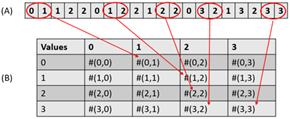Figure 2. Calculation of co-occurrencematrix

## 3.png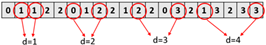Figure 3. Examples of parameter d

## 4.png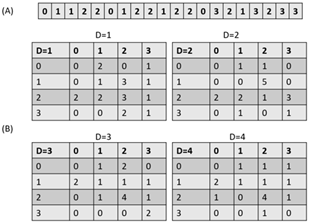Figure 4. Computing co-occurrence matrices for different distances

As can be shown in Figure 4, different Co-Occurrence Matrices are obtained for different values of d. This co-formation matrix is then normalized. Normalization is the ratio of the Co-Occurrence Matrix to the total number of pixels in a cell. Finally, signal analysis is applied by using the statistical data of the Co-Occurrence Matrix. Heralick suggested different features that can be derived from the Co-Occurrence Matrix. These features are calculated with Eqns. (2)-(19) .

Angular Second Moment

$f 1=\sum_{i=1}^{N_{g}} \sum_{j=1}^{N_{g}}\left(\frac{P(i, j)}{R}\right)^{2}$       (2)

Contrast

$f 2=\sum_{n=0}^{N_{g}-1} n^{2}\left\{\sum_{|i-j|}\left(\frac{P(i, j)}{R}\right)\right\}$      (3)

Correlation

$f 3=\frac{\sum_{i=1}^{N_{g}} \sum_{j=1}^{N_{g}}[i j P(i, j) / R]-\mu_{x} \mu_{y}}{\delta_{x} \delta_{y}}$       (4)

Variance

$f 4=\sum_{i=1}^{N_{g}} \sum_{j=1}^{N_{g}}(i-\mu)^{2} P(i, j)$      (5)

Inverse Different Moment

$f 5=\sum_{i=1}^{N_{g}} \sum_{j=1}^{N_{g}} \frac{P(i, j)}{1+(i+j)^{2}}$       (6)

Sum Average

$f 6=\sum_{i=2}^{2 N_{g}} i P_{x+y}(i)$      (7)

Sum Variance

$f 7=\sum_{i=2}^{2 N_{g}}\left(i-f_{8}\right)^{2} P_{x+y}(i)$       (8)

Sum Entropy

$f 8=-\sum_{i=2}^{2 N_{g}} P_{x+y}(i) \log \left\{P_{x+y}(i)\right\}$      (9)

Entropy

$f 9=-\sum_{i=1}^{N_{g}} \sum_{j=1}^{N_{g}} P(i, j) \log (P(i, j))$       (10)

Difference Variance

$f 10=$ variance of $P_{x-y}$      (11)

Difference Entropy

$f 11=-\sum_{i=0}^{N_{g}-1} P_{x-y}(i) \log \left\{P_{x-y}(i)\right\}$       (12)

Information Measures of Correlation

$f 12=\frac{H X Y-H X Y 1}{\max \{H X, H Y\}}$      (13)

$f 13=(1-\exp [-2(H X Y 2-H X Y)])^{1 / 2}$      (14)

$H X Y=\sum_{i=1}^{N_{g}} \sum_{j=1}^{N_{g}} P(i, j) \log (p(i, j))$      (15)

$H X Y 1=\sum_{i=1}^{N_{g}} \sum_{j=1}^{N_{g}} P(i, j) \log \left\{p_{x}(i) p_{y}(j)\right\}$      (16)

$H X Y 2=\sum_{i=1}^{N_{g}} \sum_{j=1}^{N_{g}} P_{x}(i) P_{y}(j) \log \left\{p_{x}(i) p_{y}(j)\right\}$     (17)

Maximal Correlation Coefficient

$f 14=(\text { second largest eigenvalue of } Q)^{1 / 2}$      (18)

$Q(i, j)=\sum_{k} \frac{P(i, k) p(j, k)}{P_{x}(i) P_{y}(k)}$     (19)

Here the parameters μ_x, μ_y, δ_x, and δ_y indicate the means and standard deviation of Px and Py, respectively. In addition, HX and HY are the entropy values of Px and Py .

## 5.png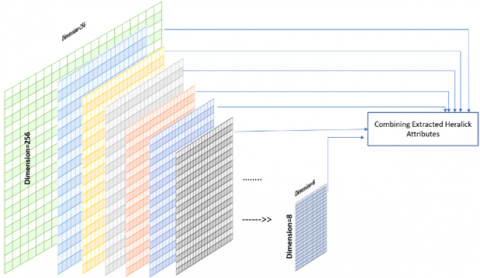Figure 5. 1D-MLGLCM method

## 6.pngFigure 6. Person identification diagram from ECG signals

4.2 One dimensional multi-layer co-occurrence matrices (1D-MLGLCM)

The 1D-MLGLCM method has been developed from the 1D-GLCM method. The 1D-GLCM method is applied to the newly obtained signals after converting the signals into different amplitude ranges. Conversion to different amplitude ranges is carried out according to the following Eqns. (20)-(21).

$A R=\{8,16,24,32, \ldots 256\}$

#anyone value from $AR$ set       (20)

$\operatorname{New} X_{i}=\operatorname{round}\left(\left[\frac{X_{i}-\operatorname{Min}(X)}{\operatorname{Max}(X)-\operatorname{Min}(X)}\right] x A R\right)$      (21)

Here AR (Amplitude Range) specifies the amplitude range into which the signal will be converted. As the number of intervals increases, the number of co-formation matrices will increase. Hence, micro-macro patterns will be easier to obtain. First, Heralick features are obtained from each co-formation matrix obtained. Then, these features are given as input to machine learning methods. The graphic of this method is given in Figure 5.

4.3 Person identification system diagram

The detailed diagram for PI from ECG signals is given in Figure 6. PI was carried out in 3 stages. The performed operations at each stage are briefly given as the following scheme.

Block 1: At this stage, ECG signals have been transformed into different amplitude ranges. Amplitude values {0-8}, {0-16}, {0-24}, {0-32}, {0- 40}, {0- 48}, {0- 56}, {0- 64}, {0- 72}, {0-80}, {0- 88}, {0- 96}, {0- 104}, {0- 112], {0- 120}, {0- 128}, {0-136}, {0-144}, {0- 152}, {0- 160}, {0- 168}, {0-176}, {0- 184}, {0- 192}, {0-200}, {0- 208}, {0- 216}, {0- 224}, {0- 232}, {0- 240}, {0- 248}, {0- 256} converted to all ranges. 32 different ranges are used. Therefore, 32 different signals were produced for each ECG signal. The conversion process is carried out with Eq. (21).

Block 2: At this stage, firstly, co-formation matrices of 32 different signals were obtained. Later, Heralick features are extracted from these co-formation matrices. In total, 448 features have been extracted.

Block 3: At the last stage, person identification was performed with different machine learning methods using all the features. Random Forest (RF), Naive Bayes (NB), K-nearest neighbor (Knn), Support Vector Machine (SVM), and Bayes Net (BN) were used as classification methods. The classification process was made according to the 10-fold cross-validation test.

4.4 Performance metrics

Accuracy, precision, recall, and f-measure were used to demonstrate the performance of the proposed angle method. These success criteria are calculated with the following Eqns. (22)-(25) [25, 26].

Accuracy $=\frac{T P+T N}{T P+T N+F P+F N}$       (22)

Precision $=T P /(T P+F P)$      (23)

Recall $=T P /(T P+F N)$       (24)

$F-Measure=2(Recall*Accuracy)/(Recall+Accuracy)$     (25)

The meaning of abbreviations in these equations is that T is true, F is false, P is positive, and N is negative. Thus, for instance, TP is the number of correctly (proper) classified positive examples; FN indicates the number of incorrectly (false) classified negative samples.

Accuracy: It is the most popular and simple performance metric to determine the success rate of a model, and this rate is defined as the ratio of the number of correctly classified (True Positive (TP) + True Negative (TN)) examples to the total number of samples (True Positive (TP) + True Negative (TN) + False Positive (FP) + False Negative (FN)).The meaning of abbreviations in these equations is that T is true, F is false, P is positive, and N is negative. Thus, for instance, TP is the number of correctly (proper) classified positive examples; FN indicates the number of incorrectly (false) classified negative samples.

Precision: It provides the degree of certainty regarding outcome of the classifier. It is the number of positively labeled samples (TP) to the total samples classified as positive (TP + FP).

Recall: The ratio of positively labeled samples (TP) to the number of genuinely positive samples (TP + FN).

F-Measure: It is calculated using precision and recall metrics. It is used to optimize the system towards precision or sensitivity.

5. Results

Each ECG signal is divided into different scales of partitions to obtain co-occurrence matrices. The scales are {0-8}, {0-16}, {0-24}, {0-32}, {0- 40}, {0- 48}, {0- 56}, {0- 64}, {0- 72}, {0-80}, {0- 88}, {0- 96}, {0- 104}, {0- 112], {0- 120}, {0- 128}, {0-136}, {0-144}, {0- 152}, {0- 160}, {0- 168}, {0-176}, {0- 184}, {0- 192}, {0-200}, {0- 208}, {0- 216}, {0- 224}, {0- 232}, {0- 240}, {0- 248}, {0- 256}. There are 32 partitions for each signal that can be considered as new signals. Figure 7 illustrates some of the new signals that are obtained from the original ECG signal.

As shown in Figure 7, there is no change in the signal structure after the partition process. Instead, each new signal is used to generate a corresponding co-occurrence matrix. Those matrices are necessary to extract 14 Heralick features for each signal, meaning 32x14=488 total number of features. These features are fed to different machine learning algorithms to classify subjects in the dataset. The features are extracted from 33 subjects that have the maximum number of measurements. The success rates of the algorithms are presented in Table 1.

SVM achieves the highest accuracy rate of 91.0151%, and NB obtains the lowest accuracy rate, which is 76.8861%, according to Table 1. Therefore, achieved success rates can be acceptable for classification. Person identification is realized with a total of 448 features. However, we observed that some of the features are inefficient in classification. On the other hand, excessive numbers of features increase the computation cost. Therefore, we deployed a method called Correlation-based Feature Selection (CfsSubset)  to eliminate inefficient features and select the most weighted features for the machine learning algorithms. Sixty-seven efficient features are selected after using the CfsSubsset method. Table 2 shows the performance evaluation of machine learning algorithms that use these efficient features.

## 7.png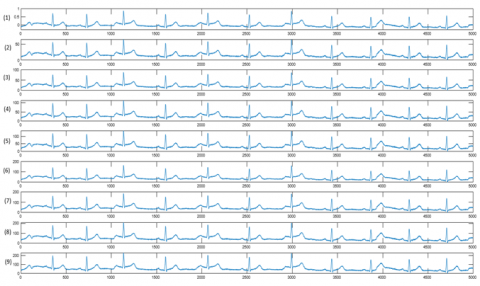Figure 7. Generated signals with different scales. (1) Original signal, (2-9) represents those partitions: {0-64}, {0-88}, {0-112}, {0-136}, {0-160}, {0-192}, {0-232}, {0-256}

Table 1. Evaluation of the algorithms

 Machine Learning Accuracy Precision Recall F-Measure SVM 91.0151 0.911 0.910 0.909 RF 89.4376 0.895 0.894 0.894 NB 86.8861 0.871 0.869 0.858 Knn 87.9287 0.882 0.879 0.879 BN 90.0412 0.921 0.900 0.903

Table 2. Success rates after feature selection

 Machine Learning Accuracy Precision Recall F-Measure SVM 87.1056 0.878 0.871 0.866 RF 88.6831 0.888 0.887 0.886 NB 90.4527 0.908 0.905 0.902 Knn 89.6433 0.898 0.896 0.896 BN 92.6475 0.942 0.926 0.929

## 8.png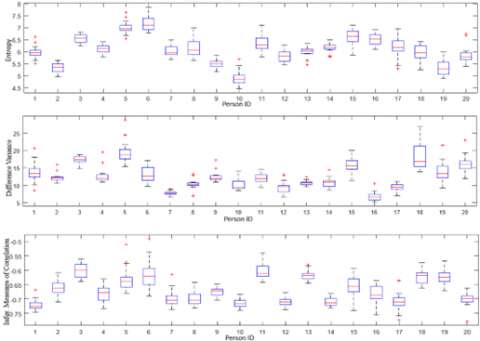Figure 8. Boxplot plots of the best three features effective in classification

SVM achieves 87.1% accuracy, which is below the previous result. However, BN's accuracy rate increased to 92.6475%. These results show that the feature selection process has a good impact on BN for this particular problem. To evaluate the effect of ECG signals on person identification tasks, a Hearlick feature extraction method is deployed. In addition to the feature extraction method, SVM is selected for the classification task. The evaluation results are shown in Table 3.

The highest success rate, 74.252%, is acquired with entropy, according to Table 3. The second best feature is Difference Variance. It will increase the success rate of person recognition if all the features in Table 3 are used together.

The top 3 features belonging to 20 subjects are presented in Figure 8 as box graphics. The variety of distribution of features according to subjects can be viewed in the figure.

The parameter d in the 1D-GLCM method represents distance. This distance is a measured neighborhood between co-occurrence matrices. It is thought that this distance is effective in acquiring micro-macro patterns. The accuracy rates with varying the distance d are shown in Table 4. SVM is selected as a classification method here.

Table 3. Success rates according to the features

 The feature Accuracy Angular Second Moment 70.9602 Contrast 57.9973 Correlation 67.4623 Variance 64.513 Inverse Different Moment 62.3182 Sum Average 61.9753 Sum Variance 70.3429 Sum Entropy 69.177 Entropy 74.2524 Difference Variance 73.4294 Difference Entropy 55.5281 Information Measures of Correlation 72.0576 Maximal Correlation Coefficient 41.0562

Table 4. Accuracy rates with different d values

 The distance value Accuracy d=1 91.015 d=2 93.414 d=3 89.511 d=4 88.694

Table 5. Identification of time domain and frequency domain

 Models 1D-MLGLCM Time Domain Frequency Domain BN 92.6475 74.399 66.634 SVM (d=2) 93.414 81.0096 76.280 RF 88.6831 83.0529 71.899 NB 90.4527 71.1538 67.019 Knn 89.6433 78.966 65.552

According to Table 4, the highest accuracy of 93.414% is obtained with distance d=2. The optimal distance value is acquired heuristically. However, high success rates are obtained with other closed values too. To test the efficiency of 1D-MLGLCM method on ECG signals for person detection, the features of the same signals are compared in the time and frequency domain.

We obtained some features such as min, max, mean, median, energy, and contrast in the time and frequency domain, entropy, correlation, and variance coefficients. Those features are used on an SVM model for the classification process. The success rates are presented in Table 5.

According to Table 5, the 1D-MLGLCM feature extraction method achieved the highest success rate compared to time and frequency domain features. Table 6 shows a benchmark of different methods in the literature of person recognition problems using ECG signals. According to this Table, the proposed method has achieved an acceptable success rate compared to other methods.

Table 6. Comparison of the results with the literature

 Reference Method Dataset Success  Autocorrelation ECG data of 56 people %96.2  Periodicity transform and NN on Euclidean distance ECG data of 52 people %92.3  MP, SVM ECG data of 20 people %95.3  Fisher Linear Discriminant Analysis (FLDA) ECG data of 10 people %97  Hadamard Transform (HT) ECG data of 18 people %97  Non-fiducial-based approach, KPCA, and SVM ECG data of 52 people %94  Autocorrelation and discrete cosine transform (AC/DCT) ECG data of 47 people %97  Diffusion maps algorithm and the Scattering Transform ECG data of 90 people %97  Root mean square value, correlation dimension, Lyapunov exponent, SVM ECG data of 26 people %80  MP-based parameters, PNN and MP-based parameters, LDA, 2NN Physionet (ECG data of 90 people) %99  1D-local difference pattern (1D-LDP) Physionet (ECG data of 90 people) %93.33 Authors of this article 1D-MLGLCM Physionet (ECG data of 90 people) %93.414
6. Discussion

Recently, the PI problem has been a popular research area in computer science. Researchers develop several techniques to solve this problem. Retina, fingerprint, face, palm images, or speech data are widely preferred data types for person recognition systems. However, those data types might not be enough for some cases in the PI problem. Person recognizing systems that have a single sensor also has some vulnerabilities. The uncertainties for those systems can be increased depending on the number of individuals. There are many advantages of multiple data type systems over single data type systems; for instance, multiple data types can provide more subjects information. In machine learning, a large number of training data and features are vital to achieve high classification accuracy. For example, two or more individuals in a crowded environment might have similar face features under different lighting and orientations. However, if we consider physical behavior, walking, posture, and facial mimics in addition to a single face image, we can obtain higher recognizing performance. ECG's unique advantages have attracted many researchers to use this signal type to build person-recognizing systems. Especially, using ECG signals provide a significant advantage because its irreplicable nature. In this study, we designed a person-recognizing system that uses ECG signals to identify individuals. The system deploys the 1D-MLGLCM method to process ECG data. The dataset includes ECG data of 90 volunteers (46 female and 44 male) whose ages ranged from 13 to 75. ECG signal from each individual has been normalized and spliced into 32 different smaller parts. Those small parts are processed by the 1D-GLCM method to create co-occurrence matrices. These matrices are necessary to extract Heralick features. Once the features are extracted, they can be fed into RF, SVM, NB, BN, and SVM classification algorithms. The proposed technique achieves a 93.414% accuracy rate with the SVM classification method. This study proves that using ECG data to recognize individuals is a highly efficient technique. Furthermore, the proposed method can be deployed to some other smart systems for PI.

Acknowledgment

This study was performed in Siirt University Faculty of Engineering Machine Vision (MaVi) Laboratory. The authors of this article would like to thank the staff of MaVi Laboratory for their support.

References

 Biel, L., Pettersson, O., Philipson, L., Wide, P. (2001). ECG analysis: A new approach in human identification. IEEE Transactions on Instrumentation and Measurement, 50(3): 808-812. https://doi.org/10.1109/19.930458

 Israel, S.A., Irvine, J.M., Cheng, A., Wiederhold, M.D., Wiederhold, B.K. (2005). ECG to identify individuals. Pattern Recognition, 38(1): 133-142. https://doi.org/10.1016/j.patcog.2004.05.014

 Arvanaghi, R., Daneshvar, S., Seyedarabi, H., Goshvarpour, A. (2017). Fusion of ECG and ABP signals based on wavelet transform for cardiac arrhythmias classification. Computer Methods and Programs in Biomedicine, 151: 71-78. https://doi.org/10.1016/j.cmpb.2017.08.013

 Golrizkhatami, Z., Acan, A. (2018). ECG classification using three-level fusion of different feature descriptors. Expert Systems with Applications, 114: 54-64. https://doi.org/10.1016/j.eswa.2018.07.030

 Jain, A.K., Flynn, P., Ross, A.A. (2007). Handbook of Biometrics. Springer Science & Business Media.

 Boulgouris, N.V., Plataniotis, K.N., Micheli-Tzanakou, E. (2009). Biometrics: Theory, Methods, and Applications. John Wiley & Sons.

 Wang, Y., Agrafioti, F., Hatzinakos, D., Plataniotis, K.N. (2007). Analysis of human electrocardiogram for biometric recognition. EURASIP Journal on Advances in Signal Processing, 2008: 148658. https://doi.org/10.1155/2008/148658

 Goshvarpour, A., Abbasi, A., Goshvarpour, A. (2017). Do men and women have different ECG responses to sad pictures? Biomedical Signal Processing and Control, 38: 67-73. https://doi.org/10.1016/j.bspc.2017.05.006

 Goshvarpour, A., Goshvarpour, A. (2019). Gender and age classification using a new Poincare section-based feature set of ECG. Signal, Image and Video Processing, 13(3): 531-539. https://doi.org/10.1007/s11760-018-1379-5

 Khazaei, M., Raeisi, K., Goshvarpour, A., Ahmadzadeh, M. (2018). Early detection of sudden cardiac death using nonlinear analysis of heart rate variability. Biocybernetics and Biomedical Engineering, 38(4): 931-940. https://doi.org/10.1016/j.bbe.2018.06.003

 Chan, A.D., Hamdy, M.M., Badre, A., Badee, V. (2008). Wavelet distance measure for person identification using electrocardiograms. IEEE Transactions on Instrumentation and Measurement, 57(2): 248-253. https://doi.org/10.1109/TIM.2007.909996

 Pathoumvanh, S., Airphaiboon, S., Prapochanung, B., Leauhatong, T. (2013). ECG analysis for person identification. In The 6th 2013 Biomedical Engineering International Conference, Amphur Muang, Thailand, pp. 1-4. https://doi.org/10.1109/BMEiCon.2013.6687703

 Waili, T., Nor, R.M., Yaacob, H., Sidek, K., Rahman, A.W.A. (2016). A hasty approach to ECG person identification. In 2016 International Conference on Computer and Communication Engineering (ICCCE), Lumpur, Malaysia, pp. 267-271. https://doi.org/10.1109/ICCCE.2016.65

 Bassiouni, M., Khaleefa, W., El-Dahshan, E.A., Salem, A.B.M. (2016). A machine learning technique for person identification using ECG signals. International Journal of Applied Physics, 1: 37-41.

 Matveev, M., Christov, I., Krasteva, V., Bortolan, G., Simov, D., Mudrov, N., Jekova, I. (2017). Assessment of the stability of morphological ECG features and their potential for person verification/identification. MATEC Web of Conferences, 125: 02004. https://doi.org/10.1051/matecconf/201712502004

 Sulam, J., Romano, Y., Talmon, R. (2017). Dynamical system classification with diffusion embedding for ECG-based person identification. Signal Processing, 130: 403-411. https://doi.org/10.1016/j.sigpro.2016.07.026

 Brás, S., Ferreira, J.H., Soares, S.C., Pinho, A J. (2018). Biometric and emotion identification: An ECG compression based method. Frontiers in Psychology, 9: 467. https://doi.org/10.3389/fpsyg.2018.00467

 Truong, T., Graf, J., Yanushkevich, S. (2019). Hybrid score-and rank-level fusion for person identification using face and ECG data. In 2019 Eighth International Conference on Emerging Security Technologies (EST), Colchester, UK, pp. 1-6. https://doi.org/10.1109/EST.2019.8806206

 Su, K., Yang, G., Wu, B., Yang, L., Li, D., Su, P., Yin, Y. (2019). Human identification using finger vein and ECG signals. Neurocomputing, 332: 111-118. https://doi.org/10.1016/j.neucom.2018.12.015

 Benouis, M., Mostefai, L., Costen, N., Regouid, M. (2021). ECG based biometric identification using one-dimensional local difference pattern. Biomedical Signal Processing and Control, 64: 102226. https://doi.org/10.1016/j.bspc.2020.102226

 Merone, M., Soda, P., Sansone, M., Sansone, C. (2017). ECG databases for biometric systems: A systematic review. Expert Systems with Applications, 67: 189-202. https://doi.org/10.1016/j.eswa.2016.09.030

 Lugovaya, T.S. (2005). Biometric human identification based on ECG. PhysioNet.

 Haralick, R.M., Shanmugam, K. (1973). Textural features for image classification. IEEE Transactions on Systems, Man, and Cybernetics, SMC-3(6): 610-621. https://doi.org/10.1109/TSMC.1973.4309314

 Hall, M.A. (1998). Correlation-based Feature Subset Selection for Machine Learning. Hamilton, New Zealand.

 Agrafioti, F., Hatzinakos, D. (2009). ECG biometric analysis in cardiac irregularity conditions. Signal, Image and Video Processing, 3(4): 329. https://doi.org/10.1007/s11760-008-0073-4

 Agrafioti, F., Hatzinakos, D. (2010). Signal validation for cardiac biometrics. In 2010 IEEE International Conference on Acoustics, Speech and Signal Processing, Dallas, TX, USA, pp. 1734-1737. https://doi.org/10.1109/ICASSP.2010.5495461

 Zhao, Z., Yang, L. (2011). ECG identification based on matching pursuit. In 2011 4th International Conference on Biomedical Engineering and Informatics (BMEI), Shanghai, China, pp. 721-724. https://doi.org/10.1109/BMEI.2011.6098470

 Camara, C., Peris-Lopez, P., Tapiador, J.E. (2015). Human identification using compressed ECG signals. Journal of Medical Systems, 39(11): 148. https://doi.org/10.1007/s10916-015-0323-2

 Hejazi, M., Al-Haddad, S.A.R., Singh, Y.P., Hashim, S.J., Aziz, A.F.A. (2016). ECG biometric authentication based on non-fiducial approach using kernel methods. Digital Signal Processing, 52: 72-86. https://doi.org/10.1016/j.dsp.2016.02.008

 Labati, R.D., Muñoz, E., Piuri, V., Sassi, R., Scotti, F. (2019). Deep-ECG: Convolutional neural networks for ECG biometric recognition. Pattern Recognition Letters, 126: 78-85. https://doi.org/10.1016/j.patrec.2018.03.028

 Goshvarpour, A., Goshvarpour, A. (2019). Human identification using a new matching pursuit-based feature set of ECG. Computer Methods and Programs in Biomedicine, 172: 87-94. https://doi.org/10.1016/j.cmpb.2019.02.009